Project showcase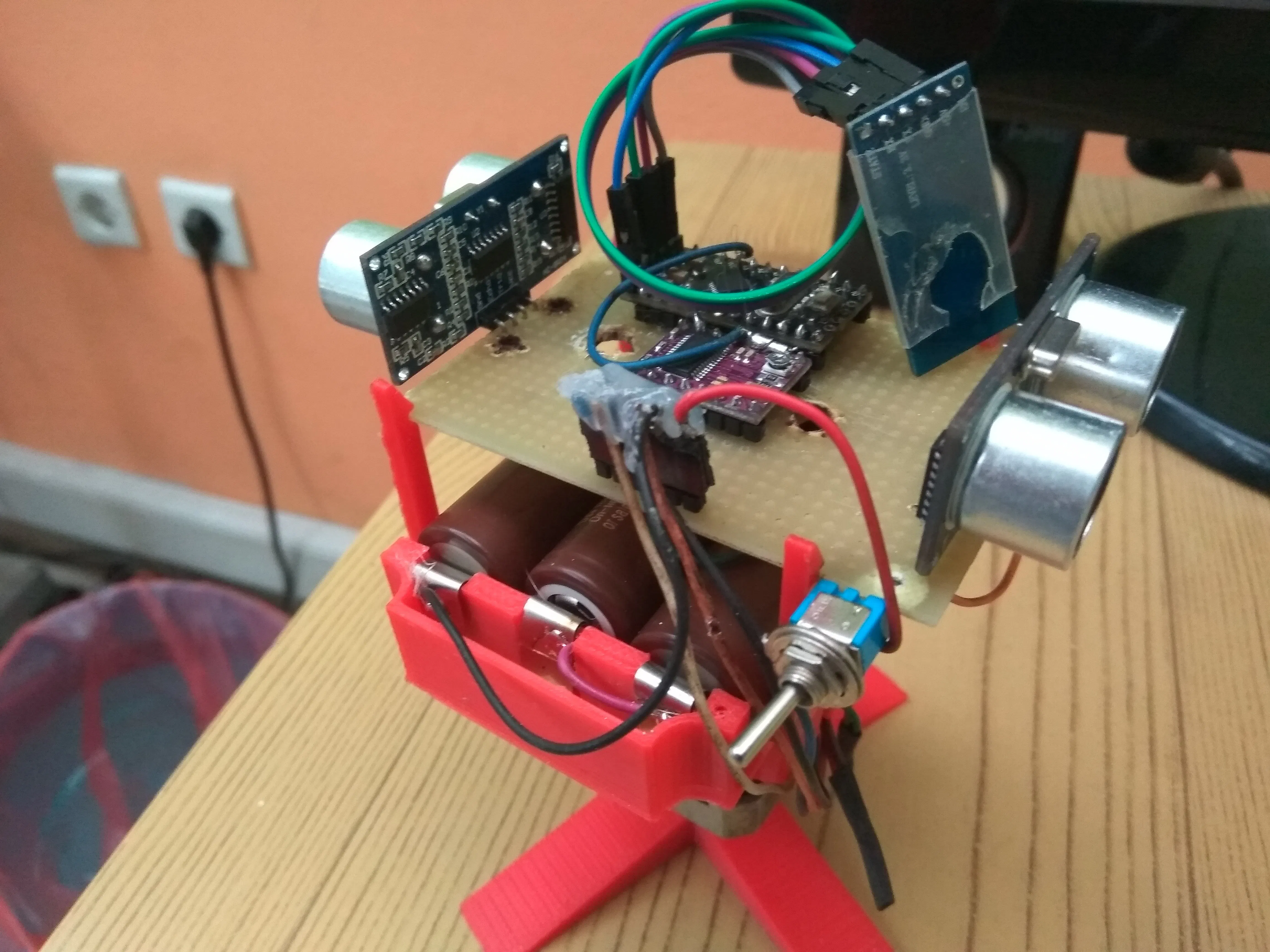# Arduino Two Ultrasonic Sensor Radar - 360 degrees Rotation

We present you the radar we created in the MicroLab lab. This radar uses 2 ultrasonic sensors and 1 motor moving continuously 360 degrees.

• 29,632 views
• 59 respects

## Apps and online services

We present you the MicroLab Arduino Radar we created in the MicroLab lab. This radar was built by:

1. The mini Arduino pro microprocessor

2. A 2-phased stepper motor of the Microcon (motor) which is set to rotate continuously 360 degrees and switches to on / off mode using a switch

3. A Bluetooth shield for Arduino to achieve microprocessor communication with the computer to transport the code

4. On / off switch

5. Three (3) Lion batteries (3000mAh each) rechargeable to supply power to the engine and microprocessor

6. The Processing 3 software for the visualization of the radar screen

7. The Arduino Software

8. Two 2 ultrasonic distance sensors for Arduino

9. One (1) DRV8825 Stepper Motor Driver Carrier, High Circle

10. Stand base made by 3d printer

We thanks Petr Zverina for making for us the stand base with his 3D printer.

## Code

##### The whole MicroLab Radar codeProcessing
```//this is the whole code running via Processing 3.3.6. (MicroLab-Greece)
//All parts tests carried out in Arduino IDE. The plastic parts are made via 3D printer
import processing.serial.*; //import library in order serial interface data
import java.awt.event.KeyEvent; //import library for reading data from serial port
import java.io.IOException;
Serial myPort;//defines serial object
//variable definition
String angle="";
String distance="";
String data="";
String noObject;
float pixsDistance;
int iAngle, iDistance;
int index1=0;
int index2=0;
PFont orcFont;

void setup() {

size (860, 860);

smooth();
myPort = new Serial(this,"COM5", 57600); //starting communication with specofic port
myPort.bufferUntil('.'); //reads data from port delimited by '.'

}

void draw() {

fill(98,245,31);
//simulation of moving line (motion and fade)
noStroke();
fill(0,4);
rect(0, 0, width, height);
fill(7,246,23);
drawLine();
drawObject();
drawText();
}

void serialEvent (Serial myPort) { //start reading data from specified port
//reads data from port delimited by (.) and assigns them in string defined variable "data"
data = data.substring(0,data.length()-1);

index1 = data.indexOf(","); //detect charachter ',' and assign it to the defined string variable 'index1'
angle= data.substring(0, index1); // read the data from position "0" to position of the variable index1 or the value of the angle, via bluetooth, sents into the Serial Port
distance= data.substring(index1+1, data.length()); //reads the data from index() from first to last (distance)
//convert stings variables into integer
iAngle = int(angle);
iDistance = int(distance);
}

pushMatrix();
translate(420,420); //moving the coordinats start
noFill();
strokeWeight(1);
stroke(255,245,255);
// draws the arc lines
arc(0,0,860,860,0,TWO_PI);
arc(0,0,720,720,0,TWO_PI);
arc(0,0,580,580,0,TWO_PI);
arc(0,0,440,440,0,TWO_PI);
arc(0,0,300,300,0,TWO_PI);
arc(0,0,160,160,0,TWO_PI);
arc(0,0,20,20,0,TWO_PI);
//darw of angle lines
line(-width/2.0,0,width/2.0,0);
popMatrix();
}
//this function draws the detected object converting the distance into pixels on screen according the angle
void drawObject() {
pushMatrix();
translate(420,420);
strokeWeight(3);
stroke(255,10,10);
pixsDistance = iDistance*2; //converting distance from cm into pixels
//with (if) we define the limit range to 2meter  (200cm) ! anyone can change the limits according to sensors specifications and drwas the line converting distance into pixel
if(iDistance<200){
}
popMatrix();
}
//this function draws the radar rotatable line
void drawLine() {
pushMatrix();
strokeWeight(6);
stroke(10,74,250);
translate(420,420);
popMatrix();
}
//function drawing text on screen nad its values taking from sensors
void drawText() {

pushMatrix();

fill(0,0,0);
noStroke();
rect(0, 1010, width, 600);
textSize(15);
fill(5,255,5);

translate(640,294);
text("30°",0,0);
resetMatrix();
translate(548,200);
text("60°",0,0);
resetMatrix();
translate(420,160);
text("90°",0,0);
resetMatrix();
translate(292,199);
text("120°",0,0);
resetMatrix();
translate(202,296);
text("150°",0,0);
resetMatrix();
translate(166,422);
text("180°",0,0);
resetMatrix();
translate(197,550);
text("210°",0,0);
resetMatrix();
translate(292,641);
text("240°",0,0);
resetMatrix();

translate(420,677);
text("270°",0,0);
resetMatrix();

translate(544,640);
text("300°",0,0);
resetMatrix();

translate(641,547);
text("330°",0,0);
resetMatrix();

translate(674,422);
text("360°",0,0);
resetMatrix();

translate(50,50);
textSize(17);
fill(255,103,1);

resetMatrix();

if(iDistance>200) {
noObject = "Out of Range";
}
else {
noObject = "In Range";
}
fill(0,0,0);
noStroke();
rect(0, 1010, width, 1080);
fill(26,230,32);

textSize(20);
text("Object: " + noObject, 250, 50);
text("Angle: " + iAngle +" °", 500, 50);
text("Distance: ", 650, 50);
if(iDistance<200) {
text(" " + iDistance +" cm", 750, 50);
}

popMatrix();

}
```
##### Motor sketch (code)Arduino
Defines and regulates the 2-phased motor rotation
```//This sketch defines and regulate the 2-phased stepper motor
int trigPinA =13;
int echoPinA=12;
volatile unsigned long durationA;
volatile int distanceA;
volatile uint16_t counter;

int trigPinB =11;
int echoPinB=10;
long durationB;
int distanceB;

volatile unsigned long Start;
unsigned long  timer1_counter;
void setup() {
// put your setup code here, to run once:
// Arduino initializations
Serial.begin(9600);

delay(1000);

Serial.write("AT+PIN1234");

delay(1000);
Serial.write("AT+BAUD8");

delay(1000);

Serial.end();

Serial.begin(115200);

//pinMode(2,INPUT);
pinMode(2, OUTPUT);

pinMode(3,OUTPUT);
pinMode(9,OUTPUT);
pinMode(10,INPUT);
pinMode(12,INPUT);
pinMode(11,OUTPUT);
pinMode(13,OUTPUT);
digitalWrite(2,LOW);
digitalWrite(9,LOW);
digitalWrite(2,HIGH);
//pinMode(2,OUTPUT);

// initialize timer1
noInterrupts();           // disable all interrupts
TCCR1A = 0;
TCCR1B = 0;

// Set timer1_counter to the correct value for our interrupt interval
timer1_counter = 65505;   // preload timer 65536-16MHz/256/100Hz
//timer1_counter = 64286;   // preload timer 65536-16MHz/256/50Hz
//timer1_counter = 34286;   // preload timer 65536-16MHz/256/2Hz

TCNT1 = timer1_counter;   // preload timer
TCCR1B |= (1 << CS12);    // 256 prescaler
TIMSK1 |= (1 << TOIE1);   // enable timer overflow interrupt
interrupts();             // enable all interrupts

}

ISR(TIMER1_OVF_vect)        // interrupt service routine
{
TCNT1 = timer1_counter;   // preload timer
counter++;
if (counter>=12800*2)
counter=0;
}

void loop() {

digitalWrite(trigPinA, LOW);
delayMicroseconds(2);
// Sets the trigPin on HIGH state for 10 micro seconds
digitalWrite(trigPinA, HIGH);
delayMicroseconds(10);

digitalWrite(trigPinA, LOW);
//Reads the echoPin, returns the sound wave travel time in microseconds
durationA= pulseIn(echoPinA, HIGH);
distanceA= durationA*0.029/2;
noInterrupts();
int i=(counter/2)/35.5555;
interrupts();
Serial.print(i);
Serial.print(",");
Serial.print(distanceA,DEC);
Serial.print(".");

digitalWrite(trigPinB, LOW);
delayMicroseconds(2);
// Sets the trigPin on HIGH state for 10 micro seconds
digitalWrite(trigPinB, HIGH);
delayMicroseconds(10);

digitalWrite(trigPinB, LOW);
//Reads the echoPin, returns the sound wave travel time in microseconds
durationB= pulseIn(echoPinB, HIGH);
distanceB= durationB*0.029/2;
noInterrupts();
i=(180+(counter/2)/35.5555);
if(i>360)
i=i-360;
interrupts();
Serial.print(i);
Serial.print(",");
Serial.print(distanceB,DEC);
Serial.print(".");

delay(0);

}
```

## Custom parts and enclosures

The motor has regulated for continiously rotation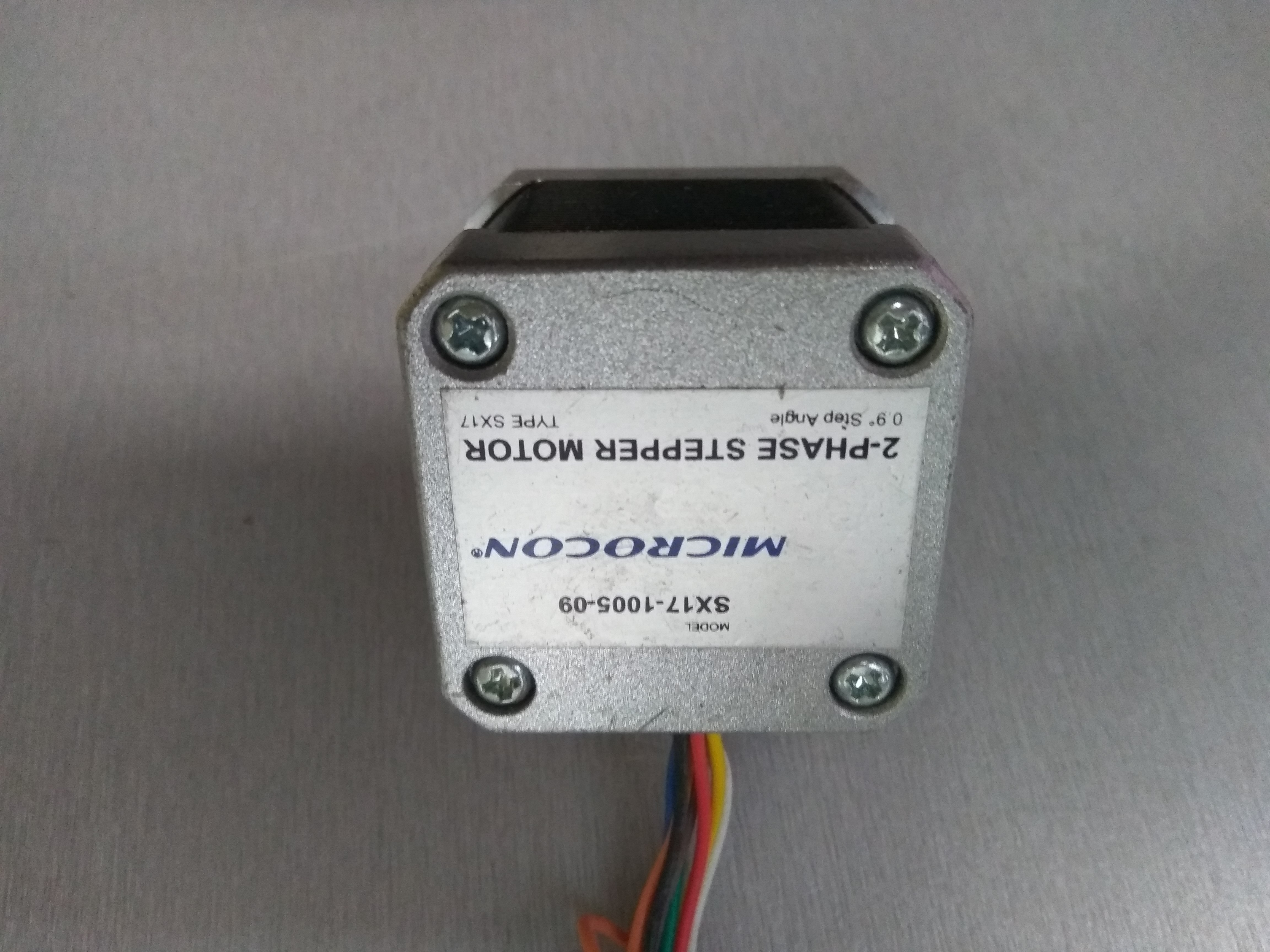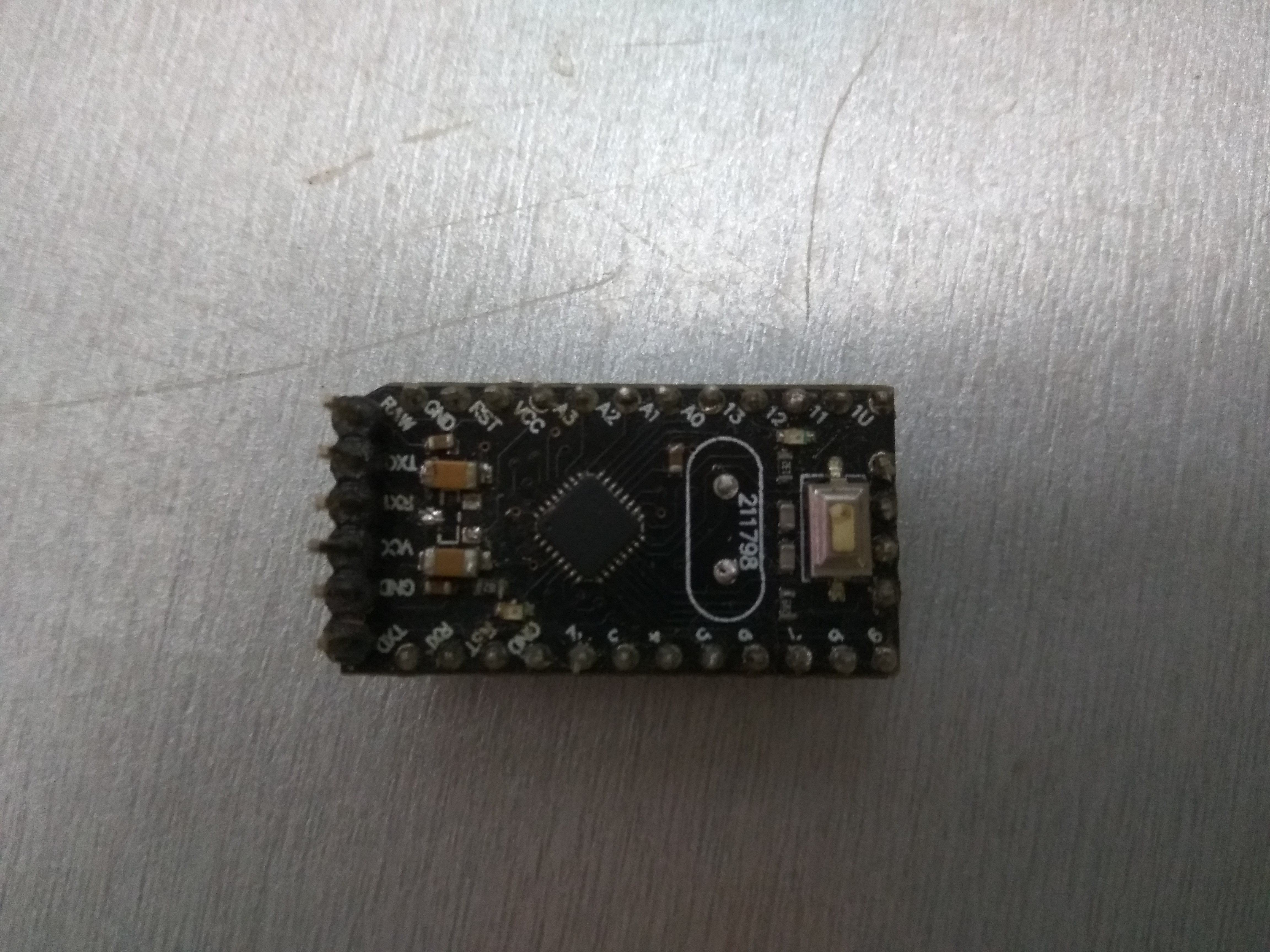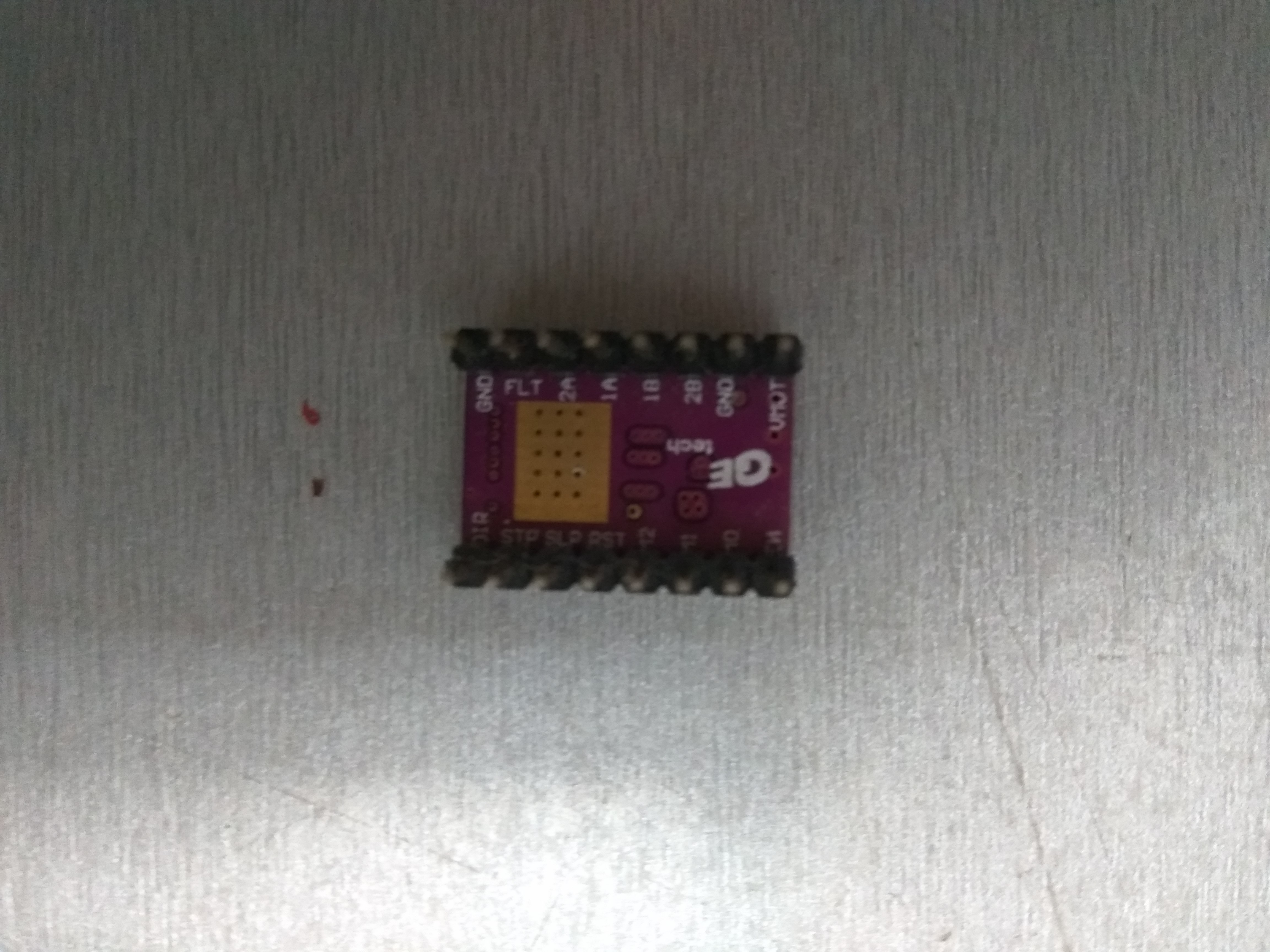All the plastic parts have made via 3D printer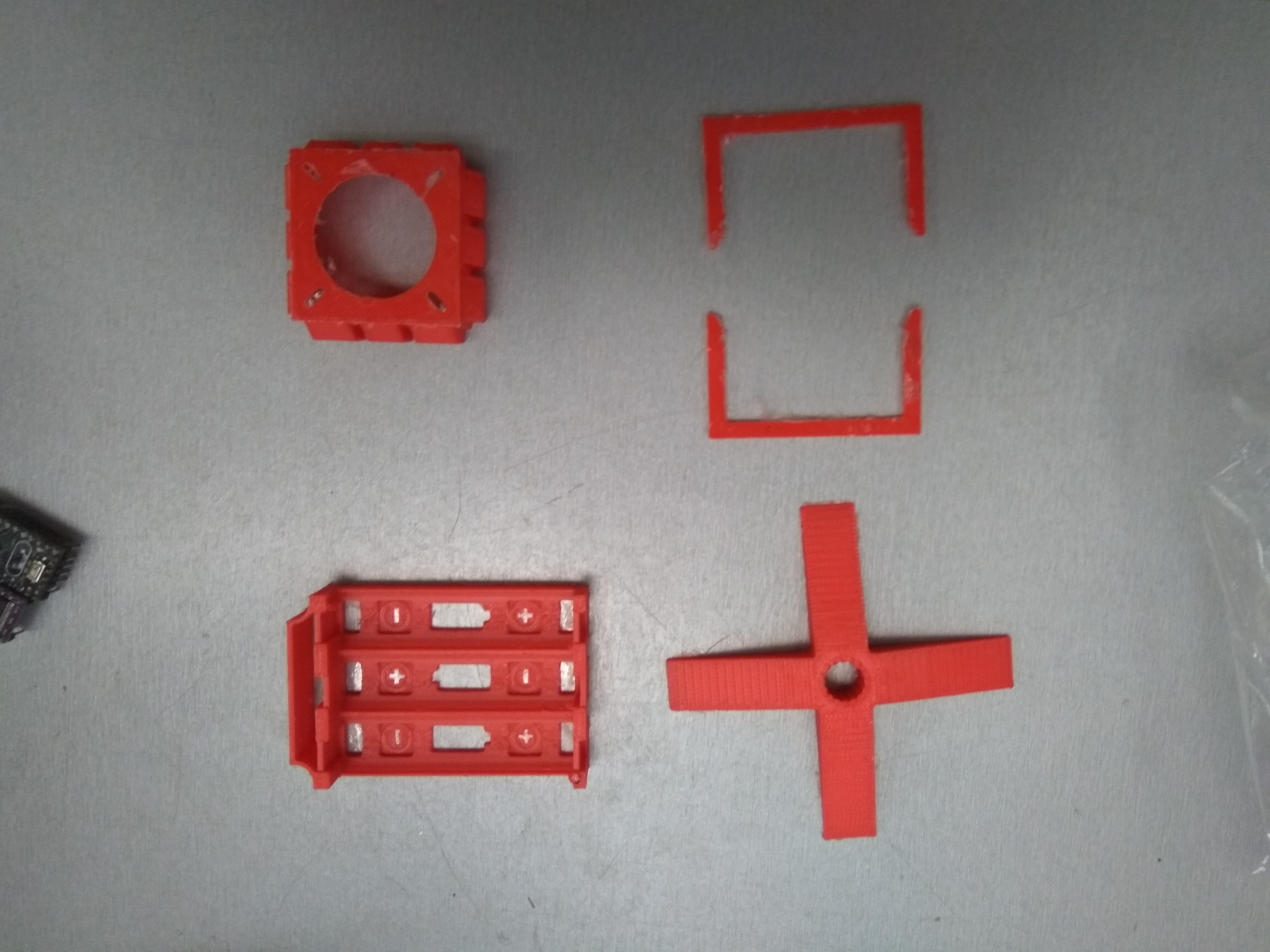The batteries are rechargeable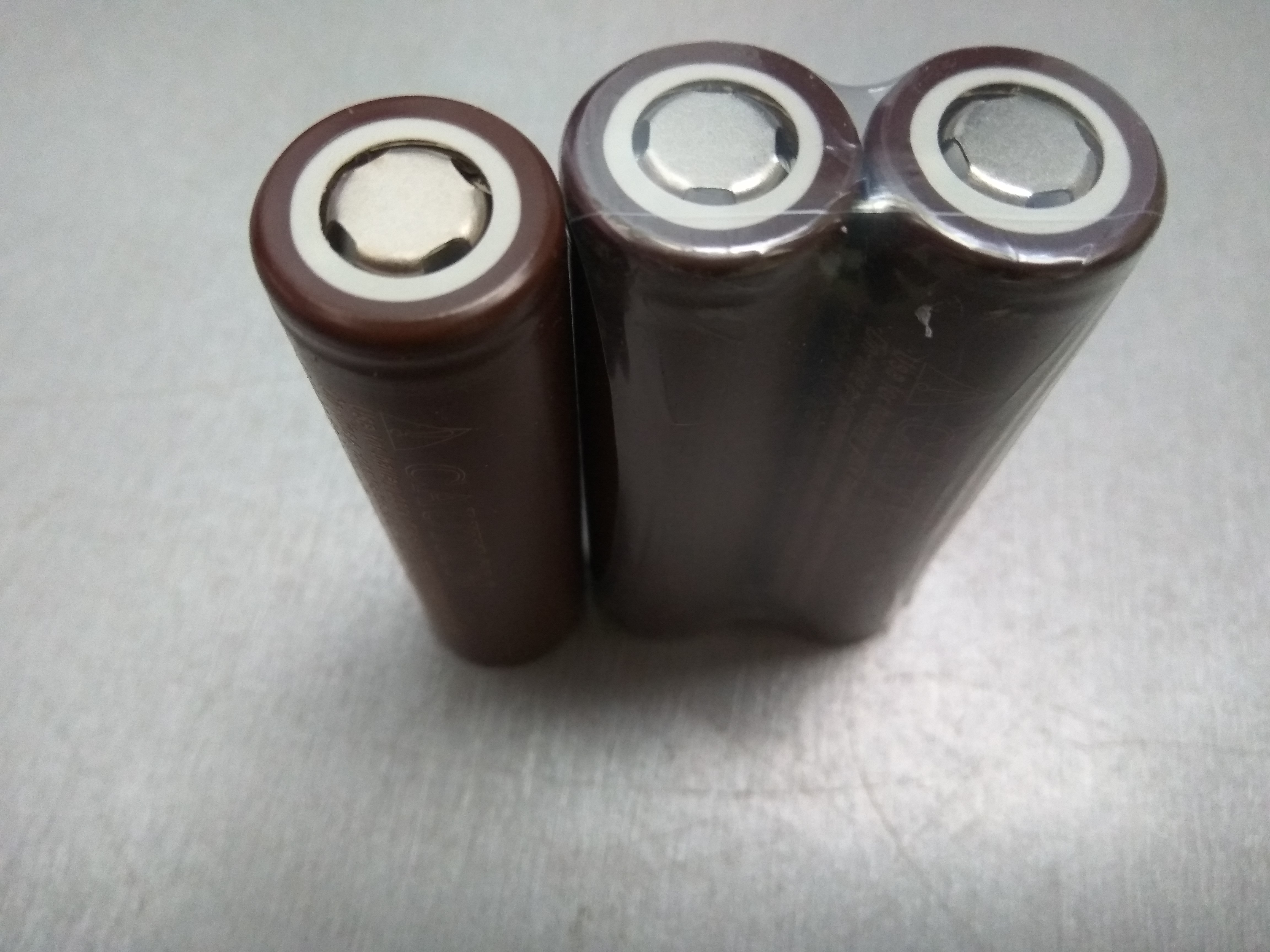Radar uses two (2) ultrasonic sensors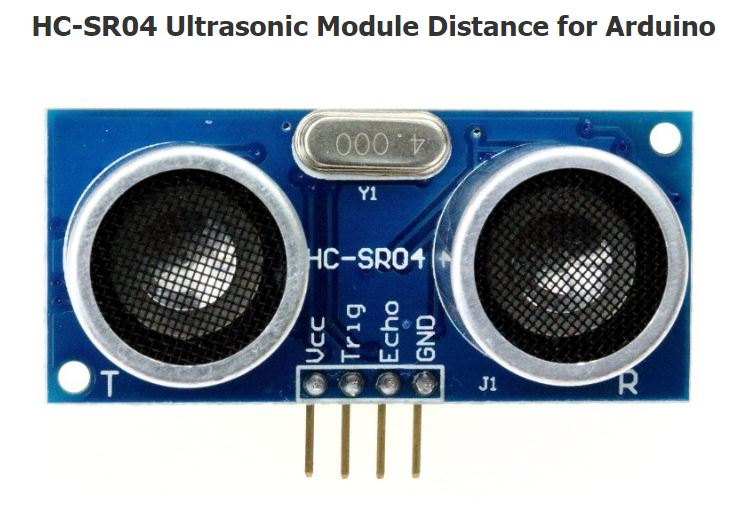Radar gets the data via bluetooth communication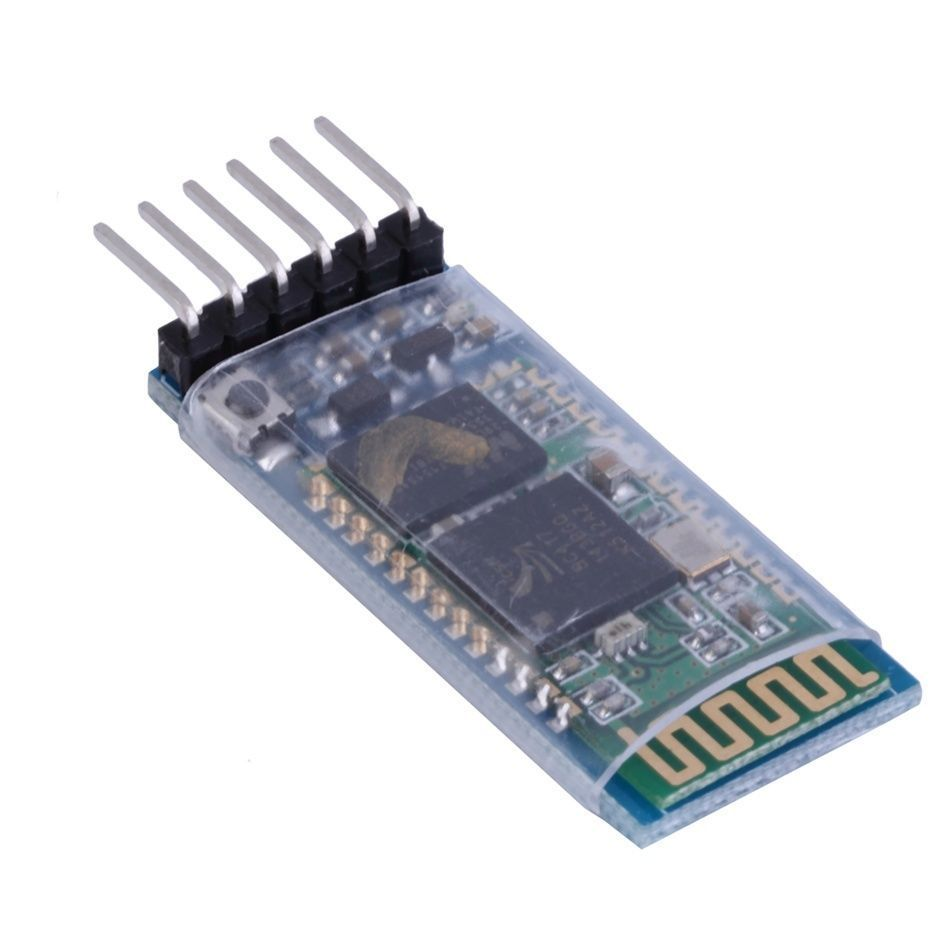#### 360 Radar (that encodes data onto an image)

Project tutorial by Alireza Karkon

• 21,207 views
• 63 respects

#### Arduino Obstacle Avoidance Robot with Ultrasonic HC-SR04

Project showcase by Jorge Rancé

• 38,129 views
• 62 respects

#### Ultrasonic Glasses for the Blind

Project tutorial by Team GardnerTech

• 47,973 views
• 120 respects

#### Ultrasonic Sensor with Alarm, LCD and Temperature

Project tutorial by MichDragstar

• 34,816 views
• 40 respects

#### Ultrasonic Sensor with LCD Display Using 433MHz

Project tutorial by mcharrison500

• 26,122 views